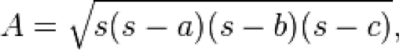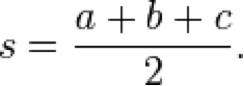It is another syntactic sugar just like where clause. By using let Expression, you can define variables and use them in other expressions.

Syntax
let <bindings> in <expression>

The variables you define in bindings section are accessed by the expression after ‘in’ part.

Suppose if a triangle has sides a, b and c. As per Herons’s formual, following formula is used to calculate area of triangle.Where s is semi perimeter.triangle.hs
`areaOfTriangle a b c = let         s = (a + b + c) / 2in        sqrt (s * (s - a) * (s - b) * (s - c))`

`Prelude> :load triangle.hs[1 of 1] Compiling Main             ( triangle.hs, interpreted )Ok, modules loaded: Main.*Main> *Main> areaOfTriangle 40 40 20387.2983346207417*Main>`

This post first appeared on Java Tutorial : Blog To Learn Java Programming, please read the originial post: here